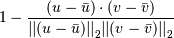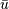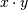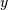# scipy.spatial.distance.correlation¶

scipy.spatial.distance.correlation(u, v)[source]

Computes the correlation distance between two 1-D arrays.

The correlation distance between u and v, is defined aswhereis the mean of the elements of u andis the dot product ofand.

Parameters: u : (N,) array_like Input array. v : (N,) array_like Input array. correlation : double The correlation distance between 1-D array u and v.

#### Previous topic

scipy.spatial.distance.cityblock

#### Next topic

scipy.spatial.distance.cosine